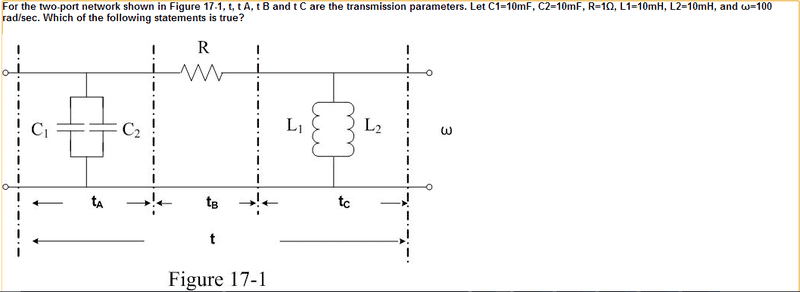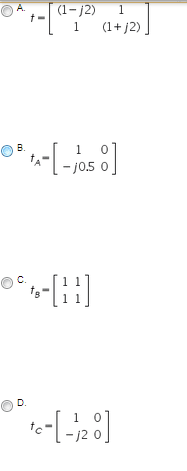# Two-port network transmission parameters?

two-port network transmission parameters??

## Homework Statementt[A B]=[t11 t12]
[C D]=[t21 t22]

## The Attempt at a Solution

so, first thing i did was find the equivalent capacitance/inductance. C(eq)=-j0.5 and L(eq)=j0.5.
using these values as I was confused by parametric equations in general i decided to do nodal equations. using V1 as the node left of resistance R, V2 as the one right of it. since in my book, they do nodal equations to check the values of y-paramter matrices.

V1 equation: (1+j2)V1+(-1)V2=0
V2 equation: (-1)V1+(1-j2)V2=0

since the t equation (choice A) had different sign values than the nodal matrix, i guessed this was wrong. choice B also looked wrong because it only had the C(eq) value, so I chose choice D because that looked similar to (1-j2)V2 in the equation for V2. i obviously know i am doing this wrong.

EDIT: looking over my notes i think this is a 't-parameter' problem. According to my teacher, the T-parameter matrices for each of the following:
Z(ohm) [T] matrix:
Code:
[1  z]
[0  1]

Y(s) [T] matrix:
Code:
[1  0]
[Y  1]

where Z and Y are the values. i am assuming Z if for resistor or any real value, Y is for imaginary part and I think the admittance. i still don't know why the matrices are structured like this, though. but if i assume this is correct, choices B, C, and D are all wrong because they don't follow the format. that leaves only A.

Last edited:

## Answers and Replies

rude man
Homework Helper
Gold Member
question 1: it says "C = 10 mF". Do they really mean 10 mF or do they mean 10 uF? It is most uncommon to express capacitance in millifarads.

Now: you need to know the two equations for the ABCD parameters in terms of i1, i2, V1 and V2 for each section t, t1 and t2. What are they?

OR: find the Z, Y or other parameters for each section and then translate to ABCD parameters.

i think i know how my teacher got t12. suppose R=3ohm, t12=V1/-I2, and -I2 is just 1V/3ohm, then 1V/-I2 would just flip it and give you the value of resistance. another way is since t12 is in units of ohms, it would just be resistance value. t21 is in units of (S) so it would just be admittance for Y(s). and i do have the equations for V1/I1/V2/I2 but i think my main problem is i don't know what values to use. it is also the only other way my book lists to find the other matrix elements.
V1=I1+0I2
V2=1I1+0I2

solve V2 equation for I1:
1I1=V2-0(I2)
so t21 and t22 should be 1 and 0. but the opposite is true.

plug in I1 into V1 equation:
V1=1(V2)+1(I2)
which gives t11=1 and t12=R..?

also, I still don't understand how to get the rest of the matrix elements for the admittance Y(s). I know t21 is in units of (S), so it should be Y, but that's as far as I get.

The Electrician
Gold Member
You already have the Y parameters. As rude man suggested, use the transformation from Y to t parameters.

You can find a table of transformations (the t parameters are also known as a parameters) here:

http://en.wikipedia.org/wiki/Two-port_network

near the bottom of the page.